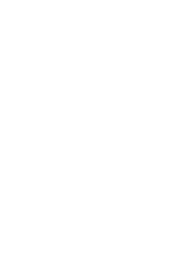http://holism.ga
Complete knowledge Graph (Cross-disciplinary Polytope Δ16)Interdisciplinary matricesCommentLaTeX CodeR codeQR codeReferencesContactMiscShow as Latex PDF

# Interdisciplinary matrices

The interactions between disciplines can be geometrically conceptualised as a cross-polytope, viz., each discipline is a node in the polytope.

An n-dimensional cross-polytope (synonymously referred to as “orthoplex”) can be defined as the closed unit ball in the ℓ1-norm on Rn:
$\{x\in \mathbb {R} ^{n}:\|x\|_{1}\leq 1\}.$

Generically speaking, each set of k+1 orthogonal vertices corresponds to a distinct k-dimensional component which contains them. The number of k-dimensional components (vertices, edges, faces, …, facets) in an n-dimensional cross-polytope is given by the following equation:

$2^{k+1}{n \choose {k+1}}$

Typesetting in
${\LaTeX\kern .15em2_{\textstyle \varepsilon }}$

and MathJaxTM

\documentclass{article}
\usepackage{tikz}
\usetikzlibrary[topaths]
% A counter, since TikZ is not clever enough (yet) to handle
% arbitrary angle systems.
\newcount\mycount
\begin{document}
\begin{tikzpicture}[transform shape]
%the multiplication with floats is not possible. Thus I split the loop in two.
\foreach \number in {1,...,8}{
% Computer angle:
\mycount=\number
\multiply\mycount by 45
\node[draw,circle,inner sep=0.25cm] (N-\number) at (\the\mycount:5.4cm) {};
}
\foreach \number in {9,...,16}{
% Computer angle:
\mycount=\number
\multiply\mycount by 45
\node[draw,circle,inner sep=0.25cm] (N-\number) at (\the\mycount:5.4cm) {};
}
\foreach \number in {1,...,15}{
\mycount=\number
\foreach \numbera in {\the\mycount,...,16}{
\path (N-\number) edge[->,bend right=3] (N-\numbera)  edge[<-,bend
left=3] (N-\numbera);
}
}
\end{tikzpicture}
\end{document}
% A complete graph
% Author: Quintin Jean-Noël
% http://moais.imag.fr/membres/jean-noel.quintin/

#library(devtools)
#install_github("schloerke/geozoo")
#install.packages("geozoo")
cross.polytope(p = 16)
##### Arguments
p = dimension of object
##### Value
points
location of points
edges
edges of the object

References

Ash, M. G.. (1998). Gestalt psychology in German culture, 1890-1967 : holism and the quest for objectivity. Cambridge Studies in the History of Psychology

Plain numerical DOI: 10.1021/acs.joc.6b02599
DOI URL

Knuth, Donald E. (2013), “Two thousand years of combinatorics”, in Wilson, Robin; Watkins, John J., Combinatorics: Ancient and Modern, Oxford University Press, pp. 7–37, ISBN 0191630624.

Rosenberg, E., & Zilber-Rosenberg, I.. (2011). Symbiosis and development: The hologenome concept. Birth Defects Research Part C – Embryo Today: Reviews

Plain numerical DOI: 10.1002/bdrc.20196
DOI URL

Rosenberg, E., & Zilber-Rosenberg, I.. (2008). Role of microorganisms in the evolution of animals and plants:The hologenome theory of evolution. F. E. M. S. Microbiol. Rev., 32, 723–735.
Rosenberg, E., Sharon, G., & Zilber-Rosenberg, I.. (2009). The hologenome theory of evolution contains Lamarckian aspects within a Darwinian framework. Environmental Microbiology, 11(12), 2959–2962.

Plain numerical DOI: 10.1111/j.1462-2920.2009.01995.x
DOI URL

Please prove you are human by selecting the Key.General Kary Mullis – full interview from 1996 (“You can’t expect the sheep to really respect the best & the brightest”)